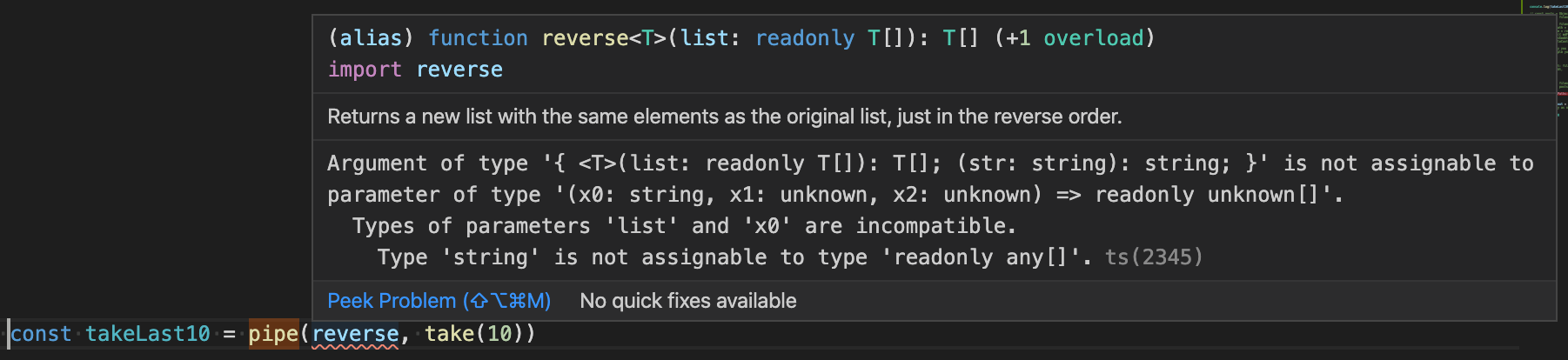SolvedDefinitelyTyped @types/ramda - Typings do not work for pipe function using R.filter

Hi. I'm trying to use R.pipe with R.filter, but I couldn't get the typing to work. But if I ignore the typings by adding // @ts-ignore the code works. I'm not asking this on StackOverflow because I'm almost sure it's a bug on the typings.

This doesn't work (the typings, the code is great, as ignoring the error part with // @ts-ignore makes it work

const searchRegexp = /\.dev\.test\.io/;
const env = 'prod';
const data = [
'https://testing.dev.test.io',
'https://testing2.local.dev.test.io',
'https://testing3.dev.test.io',
];

const values = pipe<string[], string[], string[], string[]>(
map(v => v.trim()),

/*
Below line throws:

Argument of type 'Filter<{}>' is not assignable to parameter of type '(x: string[]) => string[]'.
Type '{}[]' is not assignable to type 'string[]'.
Type '{}' is not assignable to type 'string'. (2345)
*/
filter(v => !(contains('.local.', v) && env !== 'dev')),

map(v => v.replace(searchRegexp, env === 'prod' ? '.test.com' : `.\${env}.test.io`)),
)(data.split(','));

This works (both typings & code)

const searchRegexp = /\.dev\.test\.io/;
const env = 'prod';
const data = [
'https://testing.dev.test.io',
'https://testing2.local.dev.test.io',
'https://testing3.dev.test.io',
];

const values = pipe<string[], string[], string[], string[]>(
map(v => v.trim()),
// adding this extra anonymous function here, makes everything to work
list => filter(v => !(contains('.local.', v) && env !== 'dev'), list),
map(v => v.replace(searchRegexp, env === 'prod' ? '.test.com' : `.\${env}.test.io`)),
)(data.split(','));

This is also a big problem for us, even very simple things like:

import { pipe, filter } from 'ramda';

const input = [1,2,3,4];

const output = pipe(
filter((n: number) => n % 2 === 0)
)(input);

do not compile:

Argument of type 'number[]' is not assignable to parameter of type 'Dictionary<number>'.
Index signature is missing in type 'number[]'.

The current typing for for filter is:

filter<T>(fn: (value: T) => boolean): Filter<T>;
filter<T>(fn: (value: T) => boolean, list: ReadonlyArray<T>): T[];
filter<T>(fn: (value: T) => boolean, obj: Dictionary<T>): Dictionary<T>;

For arrays the following typing is already fixing the issue:

filter<T>(fn: (value: T) => boolean, list: ReadonlyArray<T>): T[];
filter<T>(fn: (value: T) => boolean): (list: ReadonlyArray<T>) => T[];

This problem renders usage of ramda in TS pretty much unusable in some situations...

Actually, there is a type helper: <T, Kind extends 'array'>(fn: (value: T) => boolean): (list: readonly T[]) => T[];

Filter used like this should have a correct return type: pipe(filter<MyType, 'array'>(myFunction))(myArray). This is because filter is supposed to work with both arrays and objects, but typescript gets confused as a result.

I tried to make simplest pipe function:

const takeLast10 = pipe(reverse, take(10))

And got this error:It seems the issue is related to pretty much any function which can be used with more than one type, like reverse can be used for both arrays and strings.

And filter can be used with objects and arrays.

It's not excuse for ramda I suppose, but it seems to be the reason.

Here's a proposal:
We add a second type parameter to filter and reject, that specifies whether the list or object input is expected. The list is probably most commonly used so we could make that the default.

type Dictionary<T> = Record<string, T>;

type Filter<T, kind extends 'list' | 'dictionary'> = kind extends 'list' ? (list: ReadonlyArray<T>) => T[]
: kind extends 'dictionary' ? (obj: Dictionary<T>) => Dictionary<T>
: never;

declare function filter<T, kind extends 'list' | 'dictionary' = 'list'>(fn: (value: T) => boolean): Filter<T, kind>;
declare function filter<T>(fn: (value: T) => boolean, list: ReadonlyArray<T>): T[];
declare function filter<T>(fn: (value: T) => boolean, obj: Dictionary<T>): Dictionary<T>;
declare function pipe<T1, T2, R>(f1: (t: T1) => T2, f2: (t: T2) => R): (t: T1) => R;

pipe(
filter((val: string) => val.length > 2),
(x) => x.length
)(['list', 'or', 'object'])

pipe(
filter<string, 'dictionary'>((val) => val.length > 2),
(x) => x.length
)({ a: 'list', b: 'or', c: 'object' })

I have a branch for this here: https://github.com/googol/DefinitelyTyped/tree/ramda/filter-fix but I'll need to check the tests before making a PR. There seem to be tests that should catch the original problem already, but they aren't most likely making the correct assertions.

Oh yeah of course, thanks!
So for the sake of documentation, this solves our issue currently:

declare module 'ramda' {
interface Filter {
<T>(fn: (value: T) => boolean): (list: ReadonlyArray<T>) => T[];
<T>(fn: (value: T) => boolean, list: ReadonlyArray<T>): T[];
}
}